# Rounding Numbers in Excel

Excel supports several similar but different functions that round numbers.

Those functions will enable us to perform the following operations: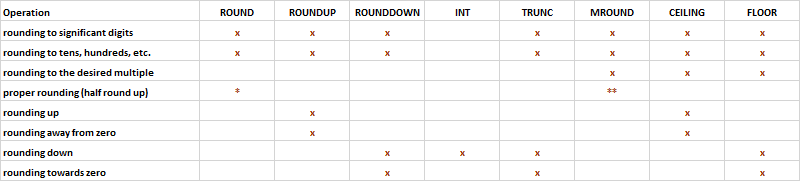* negative midpoint numbers are rounded away from zero, and not properly half-rounded up (towards positive)
**the rounding direction is undefined for midpoint numbers, both rounding up and down can happen

The ROUND function rounds a number to a specified number of digits.

The ROUND function syntax is as follows:

= ROUND ( number ; num_digits )

The ROUND function can also round a number to a specified tens, hundreds, etc. Those are specified with a negative num_digits figure, with tens being specified with -1, hundreds with -2, etc.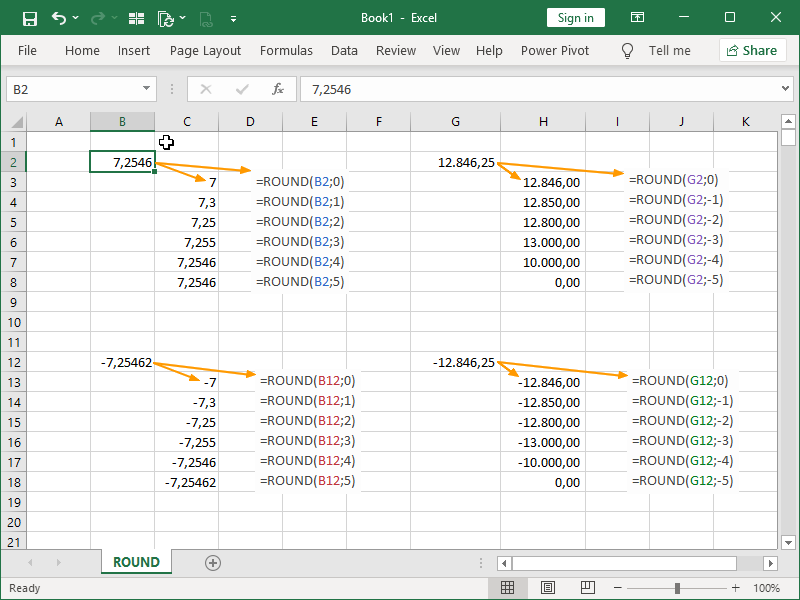The ROUNDUP function rounds a number up, away from zero, to a specified number of digits.

The ROUNDUP function syntax is as follows:

= ROUNDUP ( number ; num_digits )

The ROUNDUP function can also round a number up, to a specified tens, hundreds, etc. Those are specified with a negative num_digits figure, with tens being specified with -1, hundreds with -2, etc.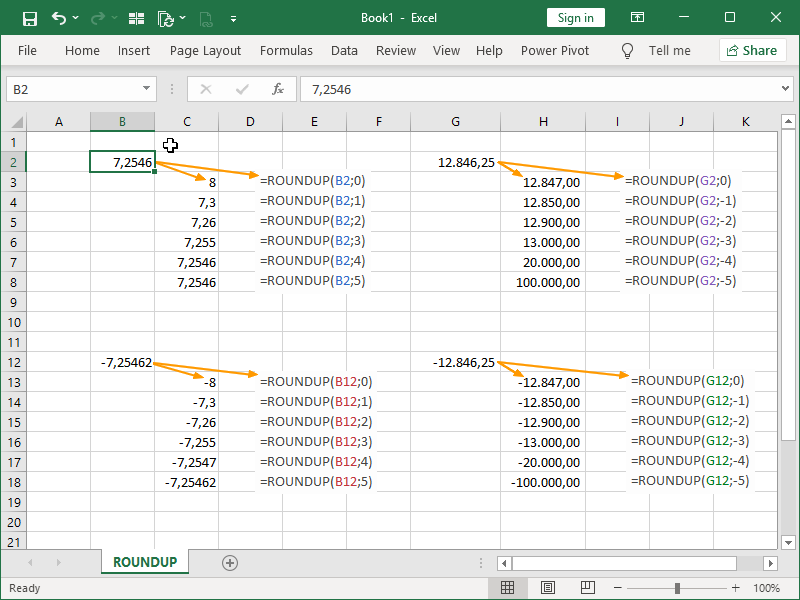The ROUNDDOWN function rounds a number down, towards zero, to a specified number of digits.

The ROUNDDOWN function syntax is as follows:

= ROUNDOWN ( number ; num_digits )

The ROUNDDOWN function can also round a number down, to a specified tens, hundreds, etc. Those are specified with a negative num_digits figure, with tens being specified with -1, hundreds with -2, etc.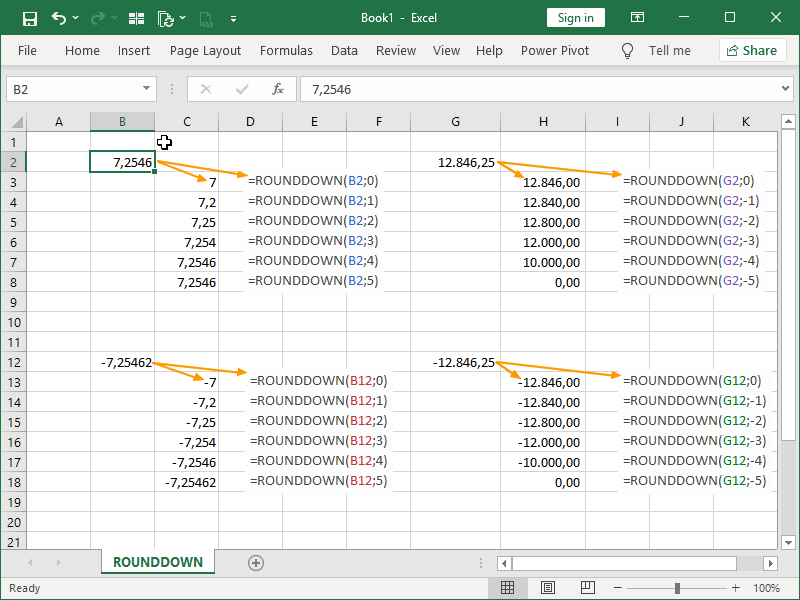The INT function rounds a number down to the nearest integer.

The INT function syntax is as follows:

= INT ( number )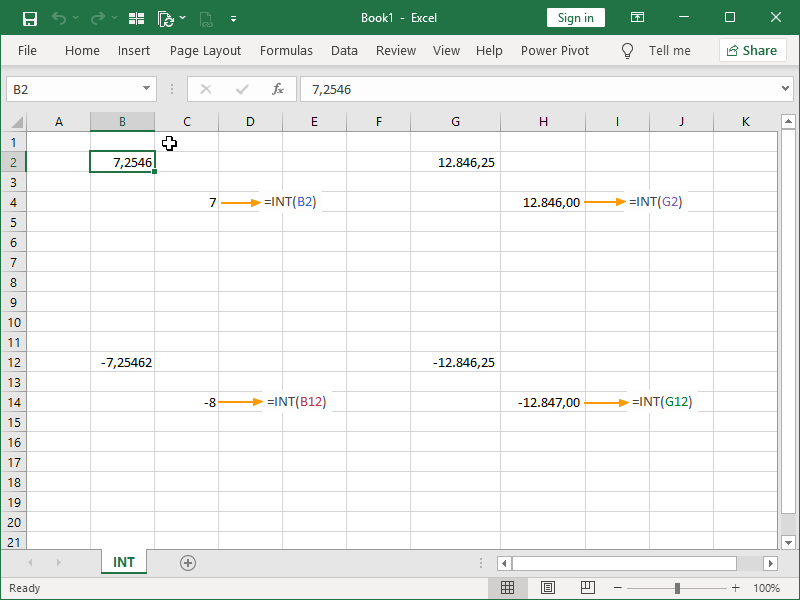The TRUNC function removes digits appearing after the specified digit, i.e. in effect, rounds a number down, towards zero.

The TRUNC function syntax is as follows:

= TRUNC ( number ; num_digits )

The TRUNC function can also round a number down, to a specified tens, hundreds, etc. Those are specified with a negative num_digits figure, with tens being specified with -1, hundreds with -2, etc.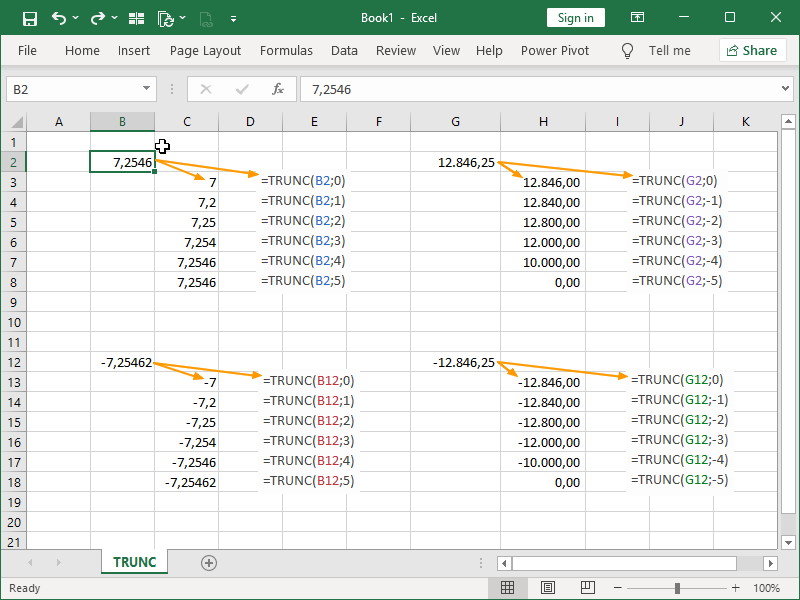The MROUND function rounds a number to the desired multiple of significance.

The MROUND function syntax is as follows:

= MROUND ( number ; multiple )

The number and multiple arguments must have the same sign.

If multiple part of the argument is zero, the MROUND function returns zero.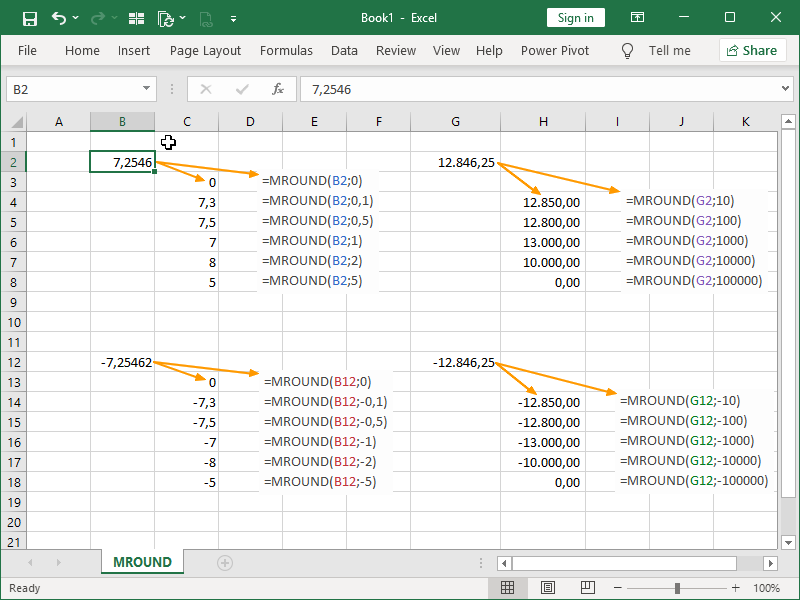The CEILING function rounds a number up, away from zero, to the specified multiple of significance.

The CEILING function syntax is as follows:

= CEILING ( number ; significance )

The number and multiple arguments must have the same sign.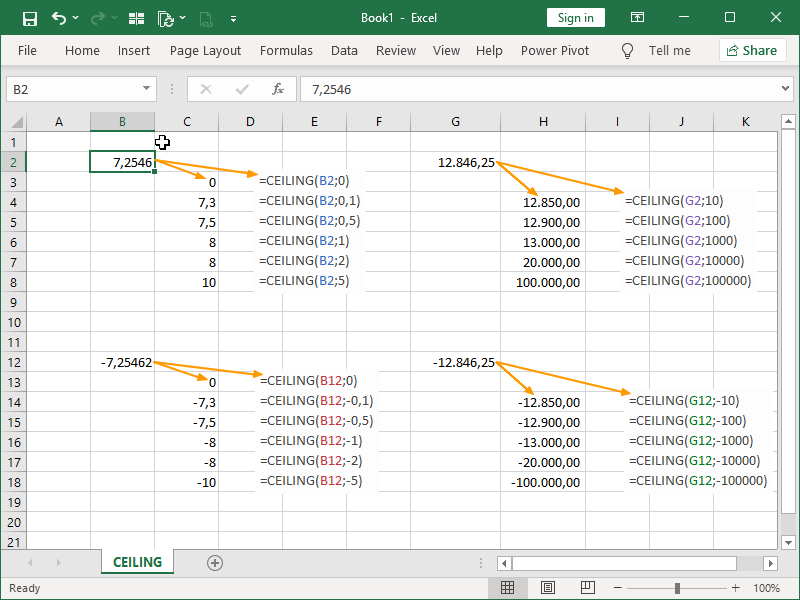The FLOOR function rounds a number down, toward zero, to the specified multiple of significance.

The FLOOR function syntax is as follows:

= FLOOR ( number ; significance )

The number and multiple arguments must have the same sign.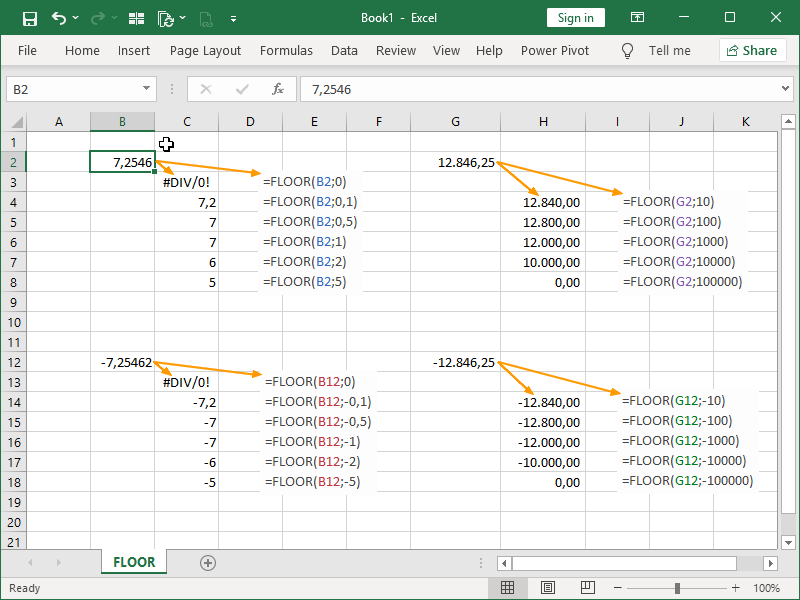Dig deeper:

Numbers in Excel